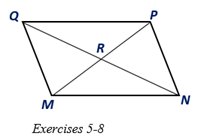Chapter 4.1, Problem 7E### Elementary Geometry for College St...

6th Edition
Daniel C. Alexander + 1 other
ISBN: 9781285195698

#### Solutions

Chapter
Section### Elementary Geometry for College St...

6th Edition
Daniel C. Alexander + 1 other
ISBN: 9781285195698
Textbook Problem
3 views

# For Exercises 5 to 8, MNPQ is a parallelogram with diagonals Q N - and M P - .If Q R = 2 x + 3 and R N = x + 7 , find QR, RN, and QN.

To determine

To find:

The lengths of the sides QR, RN, and QN in the parallelogram MNPQ.

Explanation

Given:

MNPQ is a parallelogram with diagonals QN- and MP- and QR=2x+3 and RN=x+7.

Corollary:

The diagonals of a parallelogram bisect each other.

Calculation:

In the given parallelogram MNPQ, given that the diagonals QN- and MP- and QR=2x+3 and RN=x+7.

As per the corollary that the diagonals of a parallelogram bisect each other, the diagonals QN- and MP- bisect each other at R.

So QR=RN

Substituting QR=2x+3 and RN=x+7 in QR=RN

2x+3 =x+7

2x-x

### Still sussing out bartleby?

Check out a sample textbook solution.

See a sample solution

#### The Solution to Your Study Problems

Bartleby provides explanations to thousands of textbook problems written by our experts, many with advanced degrees!

Get Started